# Which is an odd composite number

## research

### Prime number test by trial division

The method is based on the fact that a natural number n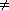1, which except 1 has no divisor d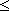n1/2 owns, is prime; is namely n = d1d2 With d1,d2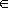N, so is d1n1/2 or d2n1/2. To see if a number n Is prime, you only need it for all prime numbers pn1/2 to test if they n share.
The effort for this prime number test is given by the number.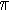(n1/2) := #{pn1/2 | p prim}

the trial divisions necessary to prove that n is a prime number. Note the well-known estimates by J. Rosser and L. Schoenfeld

For x > 17 is(x) > x/log x
and
For x > 1 is(x) < 1.25506(x/log x)
and consider, for example, a number n greater than 10100, you would have to be more than 1050/ log 1050 > 0.86·1048 Conduct trial divisions to demonstrate the primacy of n to prove: an impossible task! (log x denotes the natural logarithm of x.)

The trial division is a more exact (or more terministic) Prime test, i.e. a procedure which proves that a number n is prime.

There are also a number of methods that can determine that a number n is prime with a high probability. Such procedures are called probabilistic primality tests. One such test is the

### Fermat test.

It is based on the so-called

,,Fermat's Little Theorem". If n is a prime number, then a holds for all relatively prime numbers to n

an-1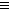1 mod n

This sentence can be used to determine whether a number n is composed. You choose a number a, (1 < a < n), and calculated an-1 mod n. Is this1, so is n composed. Is the other way around an-1 mod n = 1, it does not follow from this that n is prime. But one has for many n no evidence of the non-primacy of n found, it will be concluded that n is probably prime.

We call it a composite number n a Pseudoprime based on aif

an-11 mod n

applies. Is n a pseudoprime to the base a For all athat are coprime to n are then called nCarmichael number. There are an infinite number of Carmichael numbers. (An example of a Carmichael number is n = 561.) For this reason, the Fermat test is not suitable for practical use.

The situation is different with the following tests.

### Miller test.

The basis for this is the following tightening of Fermat's little theorem.

sentence (Miller). Let's write an odd whole number > 1 in the form-1 = 2euwith odd u, the following statements are equivalent

(i)
n is prime.
(ii)
For all numbers a that are relatively prime to n, either
(1)
au1 mod n
or there is a k in the set {0,1,...,e-1} With
(2)
a2ku-1 mod n
the test is deterministic; after a sufficient number of attempts, it can decide for every natural number whether it is prime or composite. Like the trial division, however, it is too complex for large numbers. However, it can be modified to a quick probabilistic test, the

### Miller-Rabin test

Is n a composite number and one finds one too n coprime a, for which neither (1) nor (2) for a k with 0ke-1 holds, so is a not prime Such a number is called one after Rabin Witnesses against the primacy of n. An estimate of the number of these witnesses was made by Rabin, and a simple version of his result is contained in the following

sentence (Rabin). Ben > 3 an odd composite number, then the number is deran-1that are relatively prime to n and do not bear witness to the primacy of n, at most (n-1)/4.

To apply the Miller-Rabin test to an odd number n choose any number a with 2an-1. Is the gcd (a,n)> 1, so is n composed. In the other case one calculates au,a2u,...,a2e-1u. Is a a witness against the primacy of nso it is shown that n is not prime. Let's assume that a no witness against the primacy of n is. Then the probability is that n is composed, at most 1/4. Repeating the Miller-Rabin test t-time so is n with a probability> 1- (1/4)t a prime number.

We implemented the Miller-Rabin test. He needs to test a number n the size 10100 approx. 0.062 seconds (at 1010000 approx. 3526 seconds) on a Super SPARC processor with 40MHz.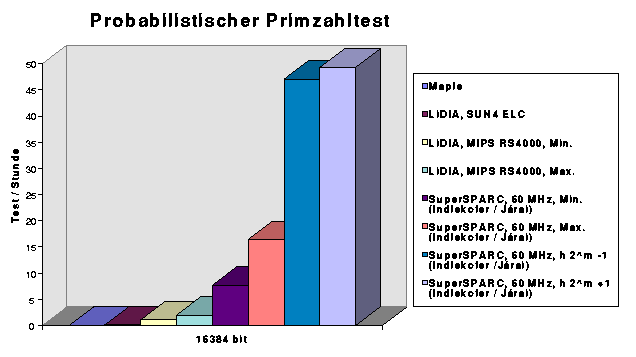literature

[1.] K.-H. Indlekofer, A. Járai, Largest known twin primes, Math. Comp. 65(1996): 427-428. MR 96d:11009

[2.] K.-H. Indlekofer, A. Járai, Some world records in computational number theory, Leaflets in Mathematics, Janus Pannonius University, Pécs, 6(1998), 49-56, ISSN 1416-0935

[3.] K.-H. Indlekofer, A. Járai, Largest known twin primes and Sophie Germain primes, Math. Comp. 68(1999): 1317-1324. MR 99k:11013